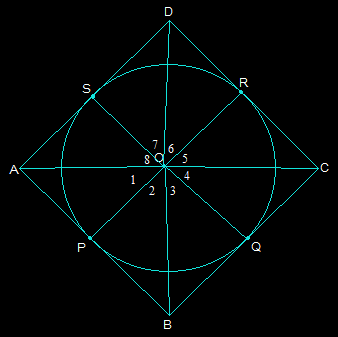Guru

# Prove that opposite sides of a quadrilateral circumscribing a circle subtend supplementary angles at the centre of the circle. Q.13

• 0

How i prove the question of class 10th ncert question from exercise 10.2 of circles chapter of question no.13, it is an important question for class 10th board exams give me the best and simple way to solve this question Prove that opposite sides of a quadrilateral circumscribing a circle subtend supplementary angles at the centre of the circle.

Share

1. First draw a quadrilateral ABCD which will circumscribe a circle with its centre O in a way that it touches the circle at point P, Q, R, and S. Now, after joining the vertices of ABCD we get the following figure:Now, consider the triangles OAP and OAS,

AP = AS (They are the tangents from the same point A)

OA = OA (It is the common side)

OP = OS (They are the radii of the circle)

So, by SSS congruency △OAP ≅ △OAS

So, ∠POA = ∠AOS

Which implies that∠1 = ∠8

Similarly, other angles will be,

∠4 = ∠5

∠2 = ∠3

∠6 = ∠7

Now by adding these angles we get,

∠1+∠2+∠3 +∠4 +∠5+∠6+∠7+∠8 = 360°

Now by rearranging,

(∠1+∠8)+(∠2+∠3)+(∠4+∠5)+(∠6+∠7) = 360°

2∠1+2∠2+2∠5+2∠6 = 360°

Taking 2 as common and solving we get,

(∠1+∠2)+(∠5+∠6) = 180°

Thus, ∠AOB+∠COD = 180°

Similarly, it can be proved that ∠BOC+∠DOA = 180°

Therefore, the opposite sides of any quadrilateral which is circumscribing a given circle will subtend supplementary angles at the center of the circle.

• 0